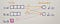# Transportation Problem: Balancing

This is part of the course “Optimization for Programmers”.

# Introduction

Transportation Simplex Method works with a balanced transportation problem. Therefore we need to learn how to make problem balanced if it is not such. And it means to cover two cases — when supply is less than demand and otherwise.

# Supply Less Than Demand

Here we can see that supply is less than demand. In such a case, we add a fake origin (d₃=10) so that supply became equal to demand. Values c₃₁, c₃₂ represent financial loss related to unmet demand.c₃₁ = 3. It can mean that the first customer will lose 3\$ with each not shipped unit.

# Demand Less Than Supply

Here we can see that demand is less than supply. In such a case we add a fake destination (s₃ = 1) so that supply became equal to demand. for unused capacity there no cost involved therefor values c₁₃ and c₂₃ are equal to 0.

# Programming

Let’s write a simple function that receives a transportation problem and returns its balanced version. When supply less than demand we also need to pass penalties(financial losses related to unmet demands).

Next part ->

Reach the next level of focus and productivity with increaser.org.